# Free-Fall Heating of Particle to High Temperatures

Harper is a manufacturer of vertical free-fall reactors for a large number of materials. As temperature increases, CAPEX becomes more significant. As a result it is necessary to use a modeling tool to correctly size the equipment for the desired production rate.

This article discusses the model, paying attention to the rigorous algorithms and the simplifying assumptions. The model takes into consideration, semi-opaque clouds (efficiency vs. efficacy), heavy particles (acceleration), families (in case sticking is anticipated), collisions between particles in varied size (or shape) and temperature dependent characteristics of the solids and gases (to account for phase changes).

The aim is to form a physically rigorous model in order simulate powder heating with a range of particle sizes. It is possible to use this as a design tool to estimate a system’s productivity.

## Limits

The assumptions made are as follows:

• The model uses a uniform particle distribution across the tube diameter
• A uniform axial gas velocity is also assumed by model, though this is in reality not the case
• Modeling the impact of falling particles on a developing velocity profile was considered highly complex at this stage
• Axial radiation is neglected in the radiation heat transfer calculation. This is not reasonable in the case of large diameter tubes
• The problem of heat transfer stops at the surface of the particle
• The relaxation time for temperature gradients R2/α may be on an identical order as the free fall time. In those cases the model must be extended along the radius of the particles

## Model

It is possible to model the problem physics to specific limits. The model is in the form of a explicit numerical solution of a system of nonlinear partial differential equations. In the case of explicit integration, any variable’s future value is a function of the present state.

While reducing the time step, the accuracy is enhanced. For specific variables it is easier to integrate directly over space steps in the place of time.

## The Force Balance

Weight = (mass of particle - mass of displaced fluid) x g (gravitational acceleration constant, 9.806 m/s2).

The other force is a drag from friction and pressure between the fluid(gas) and the particle because of the different velocity of the particles and the gas. The drag coefficient (CD) is a dimensionless number enabling the force and the Reynolds number to be related to each other.

Re is also dimensionless, which is the ratio of inertial to viscous forces exerted on the particle by the flow surrounding the particle. CD and the drag force, FD are related and is in the contrary direction of the relative velocity.

FD= CD AP ρ v2 / 2

Where AP is the projected area of the particle in the direction of movement

AP=πR2 for a sphere, ρ is the density of the fluid or in certain cases the fluid film surrounding the particle and v is the relative velocity between the fluid and the particle.

The Reynolds number is Re = ρ v D / μ. Where D is the diameter of the particle and μ is the fluid’s dynamic viscosity of the fluid, in certain cases the fluid film, around the particle.

Wilke’s mixing rule is used for viscosity

μmixture = Σ(yiμijΦij)

Φij, μ = 8 (1+ Mi/Mj) (1 + (μij)½ / (Mi/Mj) -¼))2

Where Mi is the molecular weight of species i. By volume, the example mixture is 80%Ar, 20% H2.

The raw material may be crystalline, crushed or spherical. The sphericity Ψ of a particle is the ratio of a sphere’s surface area with the same volume as the given particle to the particle’s surface area.

By definition, the sphericity of a sphere is 1. The shapes such as octahedra, tetrahedral and cubes that one may anticipate to find in a crushed hard material are 0.846, 0.671 and 0.806 respectively.

The sphericity is a parameter in the association between CD and Re.

CD = 24/Re (1 + 8.1716 e-4.0655 Ψ Re0.0964 + 0.5565 Ψ ) + 73.69 e- 5.0748 Ψ Re / (Re + 5.378 e6.2122 Ψ)

In the case of spherical particles the above equation is substituted by the much simpler relation:

CD = 24/Re (1 + 0.14Re0.7) valid for 0.1 < Re<1000

The sphericity changes for simulations extending to melting the particles

The acceleration, ai, is (FDi + (6π(ρ igas)Di3)g)/( 6πρ iDi3). FDi, ai, and g are vectors (F up and g down).

Buoyancy modifies the weight force. Velocity is given by :

vi (y+Δy) = vi (y) + aiΔ t. Substitute Δt = Δy/vi.

For vi approaching zero, this is unstable. vi=0 is a plugged reactor.

In order to study the impact of crowding other than the effect it may have on radiation heat transfer, collisions are counted.

The assumption is that all particles in a size-group move at the same speed and reach same temperature along the length and continuous particle size distribution is discretized.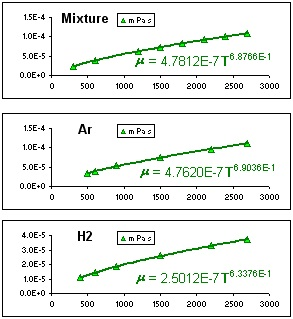Figure 1. Viscosity of Argon H2 Mixture as function of Temperature.

Image Credit: Harper

The next assumption which may not be true is a uniform gas velocity across the tube cross-section and the velocity of the particles in a particular size range is the same as all other particles in the group at that position.

However, different group particles may move at different velocities.

For example, a large particle group i moving with respect to a large number of smaller group j particles on an average will collide with the volumetric number density of j particles times the volume swept out by the i particle in a specific time increment Δt. The length increment is Δy. Hence the time step is Δy/vi (different for each particle group).

With regards to the collision with j group particles, the volume swept by the i particles are:

Vc,ij = π/4(Di + Dj ) 2Δ t|vj- Vi| = π/4(Di + Dj)2 |vj - vi| Δy/ vi.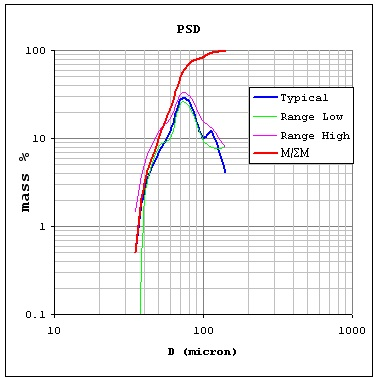Figure 2. Continuous Particle Size Distribution.

Image Credit: Harper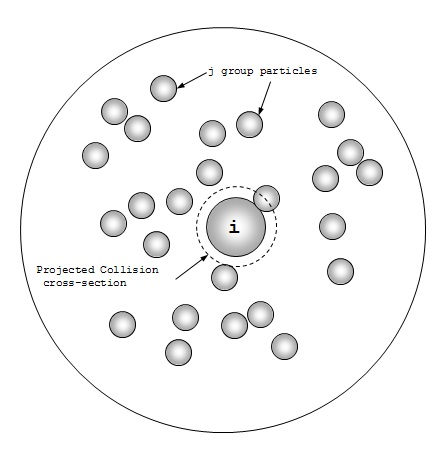Figure 3a. Centerline Cross Section.

Image Credit: Harper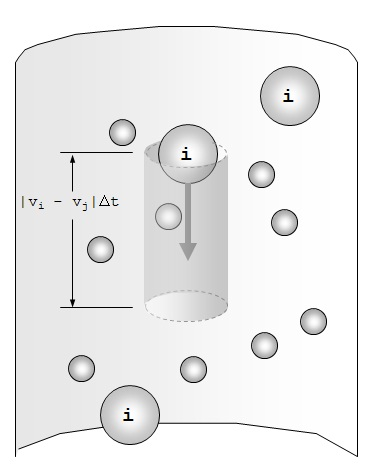Image Credit: Harper

The volumetric number density of particles of Group j, η j is the number, ΔtNj divided by the volume particles move in a time step Δt.

η j = Δt m Xj / (Δt vj π/4 Dt2) = Nj / (vj π/4 Dt2)         (1)

Nj = (m Xi / (6πρ jDj3))

Where m is the total mass rate (production rate),

Xj is the fraction of m in group j, 6πρ iDj3 is the particle mass of the j group

Δ t Nj is the number of j particles that move across any horizontal plane in the time Δt the volume of the tube over length Δt vi is (Δt vi π Dt2 / 4) is.

The average collision number that single i particles have with j group particles along length increment Δy is, therefore,

VC, ij x η j = π/4(Di+Dj)2|vj- vi| Δy/ vi Nj / (vj π/4 Dt2) = Nj (Di +Dj)2|vj-vi|/(Dt2 vjvi) Δy

Summing over j, the total number of collisions for one i particle over length Δy, NC,i is

NC,i = {Cij} {Nj} Δy,

where Cij = (Di + Dj)2|vj-vi|/(Dt2 vjvi) and {Nj}= {Ni}T.={0, N2, N3,...}, index 0 is the tube.

Thermal energy balance is also explicitly integrated over time.

## Black Bodies

Calculation of radiation heat transfer is done between the furnace and the particles and radiation between particles that because of their different sizes may be at varied temperatures along the free-fall length.

In the case of cylindrical coordinates the areas dA are simply Rtd αdz.

In the case of empty tube the integrand is dA1 cos(θ1) dA2 cos (θ2)/pr122. The cos(θ2) term is the projection of dA2 in the direction of dA1. It is possible to prove that cos(θi) = cos(θ2). The vector from dA1 to dA2 is r12.

Based on the number density η i, the volumetric density β is defined:

β = Σ i βi = Σi ηi π Di2 / 4, β has units of L-1 (=L2/L3).

The variation in the intensity I of collimated light is dI = -β I dx.

Integrating over a finite length L, I(L) = I(0) e-βL. A new integrand is obtained by applying the shading of the particles to the radiation view factor calculation

exp(-β|r12|)(|r12•R1|/ |r12| |R1|)² (1/πr12²) Rt1dz1 Rt2dz2.

A Monte Carlo style algorithm was integrated to this and the result was verified against published values. The points are generated using r/R intercepts from the referenced graph. The calculation of the curve is done with b=0 at varied L/R:

A different cloud density measure is used which is related to β by this simple relation:

A tube/A total = π Dt dy / (π Dt dy + π/4 Dt2 dy x 4β) = 1/(1 + βDt).

A little manipulation helps obtain a relationship where f(Ftt) and g(A tube/A total) satisfy f(1) =g(1) = 0.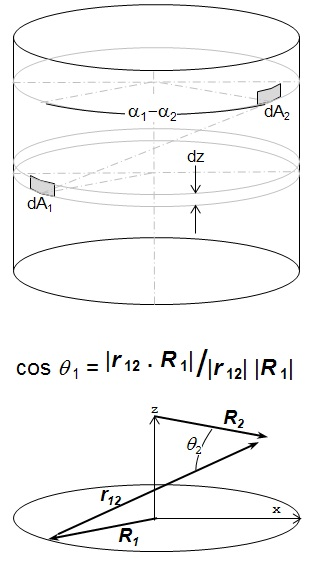Figure 4. Cylindrical Geometry for Integration.

Image Credit: Harper

By definition, all views from any giving body sum up to one.

Σ j Fij = 1                 (2)

The view which body 1 has of body 2, F12 is associated with the view body 2 has of body 1.

A1F12 = A2F21 or F21 = (A1/A2) F12         (3)

For determining heat transfer, each group by particle size is treated as one body. The assumption is that particle distribution is uniform across the cross section of the tube. Based on their areas, the view from the tube to the particles is distributed among the particle sets The total particle area is Σ j≠i Aj.

The area fraction of a single group, i, is Aij≠i Aj.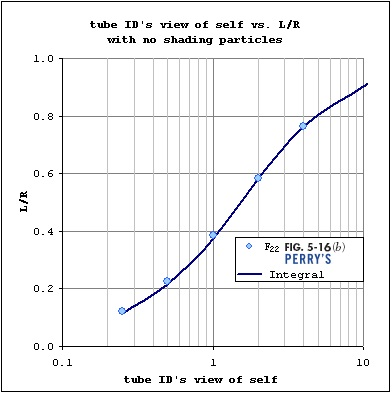Figure 5. View Factor of Tube on Tube.

Image Credit: Harper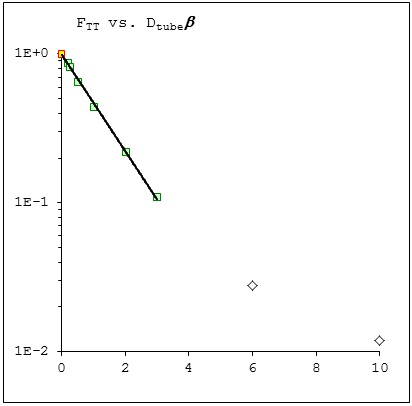Figure 6. View Factor as a Function of Tube Diameter.

Image Credit: Harper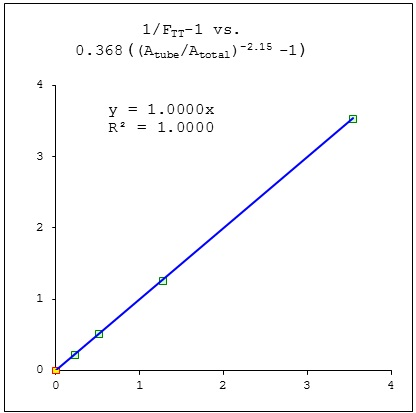Figure 7. Linear Fit of View Factor to the Fractional Area.

Image Credit: Harper

Ftp = 1 – Ftt

from equation 2. To simplify the subscripts let F ti = F tp, i,

At F ti = A tF tp Aij≠1 Aj,

by distribution over areas argument

Fpt = Ftp Atj Aj,

from equation 3

Ai Fit = At F Ti = A tF tp Aij≠1 Aj,

Fpp = 1 – Fpt

from equation 2

Ai Fij = AiFpp Ajj≠1 Ak ,

note Fii ≠ 0, group i particles see each other.

Highly dense clouds of particles βDt>>1 may require this work to be extended to verify for radial gradient. The radial gradient, however was not used in the results.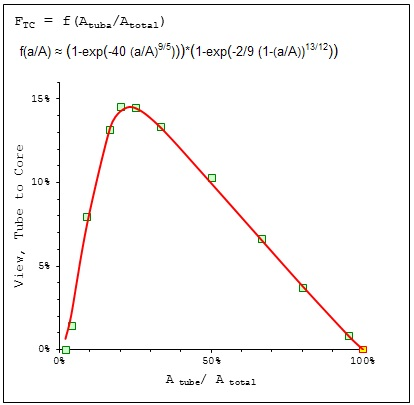Figure 8a. View Factor of Tube to the Tube's Core as a Function of Fractional Area.

Image Credit: Harper

## Grey Bodies

The above formulae are for black particles (emissivity = 1). For grey particles (emissivity: independent of wavelength but less than 1) it is important to account for reflected light.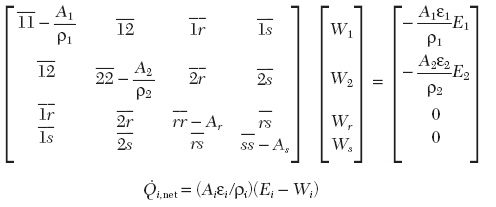Image Credit: Harper

11 with an over bar is Fractional Area shorthand for A1F11, ε1 is the emissivity of body 1 and the reflectivity is ρ1 (ρ = 1-ε, yet another ρ). Ei is the blackbody radiative flux from i, σT4 where σ is the Stefan-Boltzmann constant.

The system of equations is solved for the Wi and with this the net radiation to the surface i is obtained:

The matrix and vectors can be constructed using index vectors, i = {1, 2, ...} and j = iT

{Wi}T = {Ωij}-1i}T

Ωij = AjBiFij - δij Ai/(1- &epsiloni)

Δi = -Ai εi/(1-εi) Ti4

Aj = AiT, Ai = {A Tube, A Particle group 1, A Particle group 2…}

Bi = {Bi}: Bi=1 = 1, Bi>1 = Ai/ (Σk≠1Ak)) …

Fij = {Fij}: Fi=1, j=1= FTT, Fi>1, j=1= FTP, Fi=1, j>1= FPT, Fi>1, j>1= FPP

δij = 1 if i=j, 0 otherwise

Notes:

The “i” and “j” here include the tube (i, j = 1).

The W in the reference and here differ by a factor of σ.

The radiation heat transfer to a single particle is Qrad, i = σπDi2εi/(1- εi) (Wi – Ti4)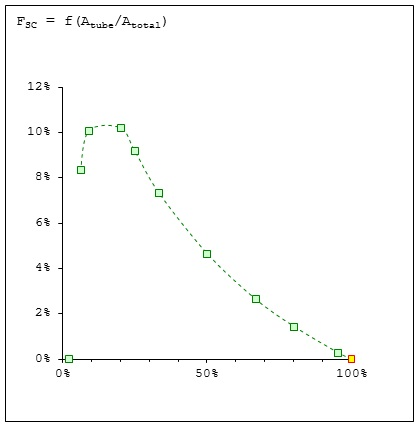Figure 8b. View Factor of Shell to the Tube's Core as a Function of Fractional Area.

Image Credit: Harper

Flow-dependent empirical correlations are used for calculating the convective heat transfer between the fluid and the particles. It is assumed that the flow is undisturbed by other particles Most simulated cases have a high volumetric loading of below a few percent. It is also assumed that the gas is non-radiating. For systems where gas is flowing, it is important to track or model the gas temperature along the length also.

In case the velocity of gas is zero there cannot be any net heat transfer to or from the gas. This is mathematically stated as the "the sum of the heats transferred to the gas is zero" or: Σi hiAi (Ti-Tg) = 0, i hi, Ai, and Ti are the local heat transfer coefficient, area and temperature of surface "i". Note, again: "i" includes the tube.

The no-net-flow gas temperature is

Tg, NF = Σi (hiAiTi)/ Σj (hiAi).

The gas allows heat transfer between different surfaces in parallel with radiative heat transfer. The process can use flowing gas in different ways. The residence time in the hot zone is extended by gas flowing up past the falling particles. Gas flowing down along with the particles can bring down the velocity range and the number of collisions.

For flowing gas, an explicit integration is more complex. The solution method which works for both extremes and the range between them is to use an integrated form:

m. g Cp,g dTg = Σi hidAi (Ti-Tg)

Where dAi = (Ai/Δy)dz, m. g and Cp,g are the mass rate and heat capacity of the gas, respectively. This can be integrated over 0

Tg(y+Δy) = Tg(y) + (Ts - Tg(y))(1-exp(-HA/ m.g Cp,g))

HA = Σi(hiAi), Ts = Si (hiAiTi)/HA.

The results do not show sensitivity to if "i" refers to the local temperature or upstream. It is more important to track heat carried by gas for high flow cases.

The correlation used to get the gas-powder heat transfer coefficient is:

Nui = 2 + 0.6Rei½ Pr1/3

Re is previously defined. The Prandtl number Pr ( = Cp μ/k) - is considered relatively constant since the thermal conductivity and viscosity of a gas show similar temperature dependence. In the example, the difference over 3000K was around 3%.

hi=Nuiki /Di, ki = kT0 (½/ (Ti+Tg)/To)kA

For cases wherein Aparticies>Atube and hshape = Nu kgas/Dshape yielding hparticle>>htube, it is possible to neglect convection to and from the tube wall.

The particle temperature as a function of time and/or distance is, in explicit numerical integration, a simple algebraic relationship:Figure 9. Velocity and Particle Count for various Particle Sizes.

Image Credit: Harper

T(t+Δt) = T(t) + Δt(net heat transfer rate to particle)/(particle mass x heat capacity)

This model allows the passage of materials through energetic phase transitions. This is mathematically captured by making the heat capacity a function of temperature. An enthalpy plot versus temperature is fit with a Cp (T). The iteration time steps need to be very small and phase temperature band wide enough so that as the element passes through the phase change, the temperature changes.

## Results

The model helps obtain graphs showing all input parameters. For example Figure 10 shows along with the temperature for each size group and the boundary conditions, the material thermodynamics, the particle size distribution, the particle velocities for each size, the collision count and the full set of input parameters.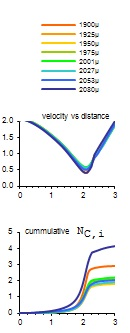Figure 10

Image Credit: Harper

Harper International is a global leader in complete thermal processing solutions and technical services essential for the production of advanced materials. From concept to commercialization, from research scale to full production line operations, Harper is perpetually on the cutting edge of the most innovative furnace and oven designs in the world. For decades, we have pioneered some of the most unique, customized systems available, with a focus on processing materials at high temperatures up to 3000°C and in non-ambient atmospheres.

Harper serves advanced, cutting-edge material markets including Fibers & Filaments, Powders, Metal Oxides, Technical Ceramics, Rare Earths, Graphene, Energy Device Materials and Nuclear Materials. Our support to these emerging industries begins in early stages of research and development, whether at corporate R&D centers, universities, government institutions, or start- ups. Harper is a partner through the entire development process assisting in the scale up and commercialization of advanced materials that will change our everyday lives.This information has been sourced, reviewed and adapted from materials provided by Harper International.

## Citations

• APA

Harper International. (2020, April 24). Free-Fall Heating of Particle to High Temperatures. AZoM. Retrieved on September 20, 2020 from https://www.azom.com/article.aspx?ArticleID=11945.

• MLA

Harper International. "Free-Fall Heating of Particle to High Temperatures". AZoM. 20 September 2020. <https://www.azom.com/article.aspx?ArticleID=11945>.

• Chicago

Harper International. "Free-Fall Heating of Particle to High Temperatures". AZoM. https://www.azom.com/article.aspx?ArticleID=11945. (accessed September 20, 2020).

• Harvard

Harper International. 2020. Free-Fall Heating of Particle to High Temperatures. AZoM, viewed 20 September 2020, https://www.azom.com/article.aspx?ArticleID=11945.

## Tell Us What You Think